Integration Double Jeopardy Integration by Integration Substitution By

• Slides: 41Integration Double Jeopardy Integration by Integration Substitution By Parts Definite Integrals Homework Exercises Potpourri 200 200 200 400 400 400 600 600 600 800 800 800Integration by Substitution: 200Integration by Substitution: 400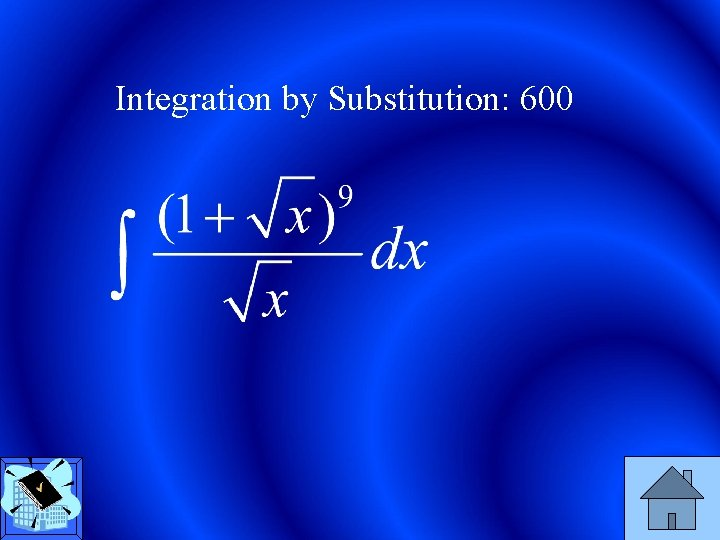Integration by Substitution: 600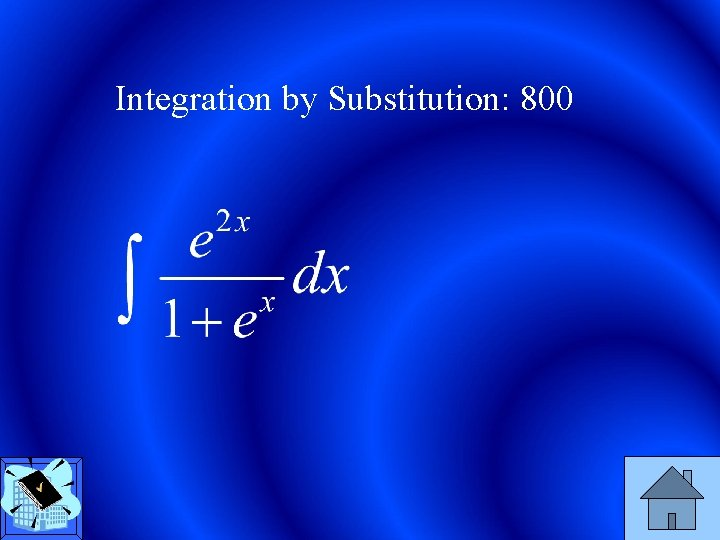Integration by Substitution: 800Integration by Parts: 200 Daily Double - 400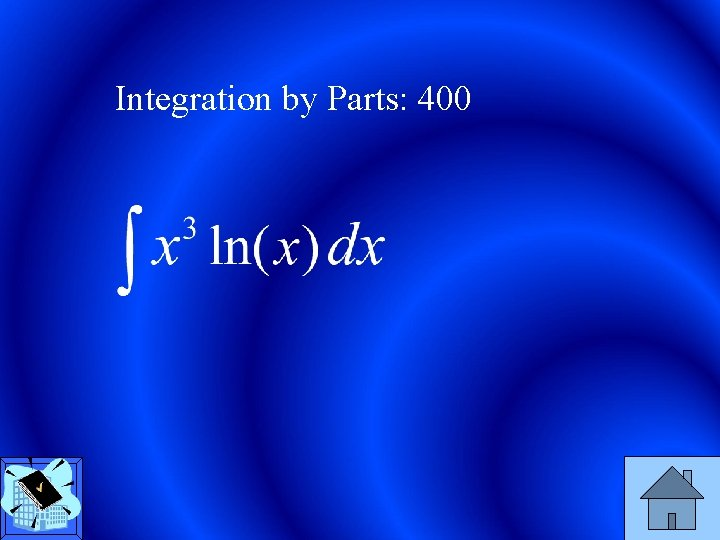Integration by Parts: 400Integration by Parts: 600Integration by Parts: 800 Daily Double - 1600Definite Integrals: 200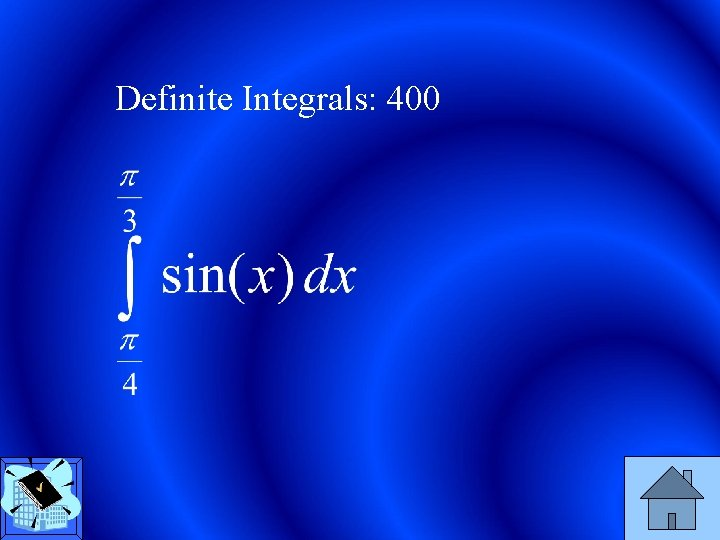Definite Integrals: 400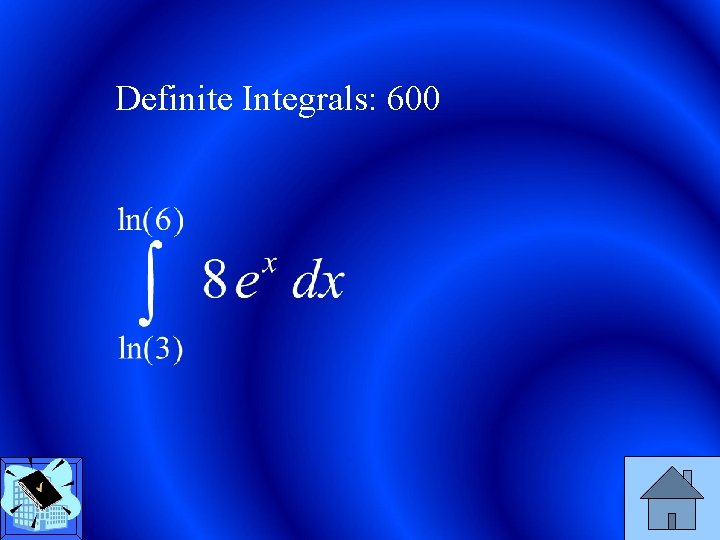Definite Integrals: 600Definite Integrals: 800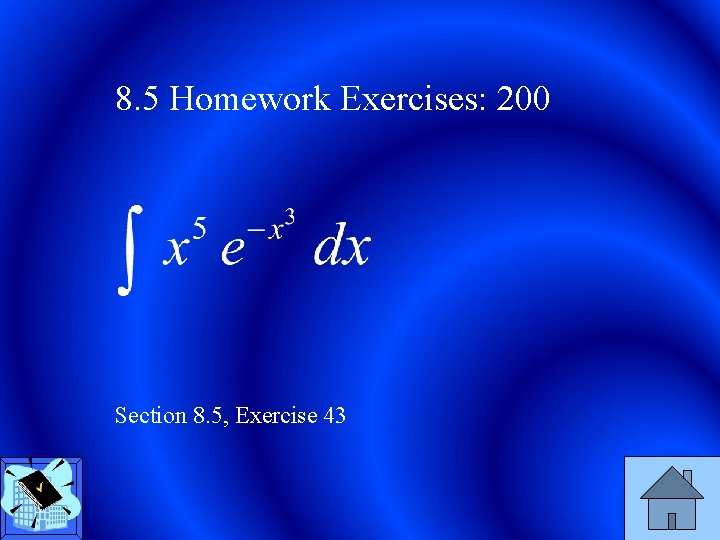8. 5 Homework Exercises: 200 Section 8. 5, Exercise 43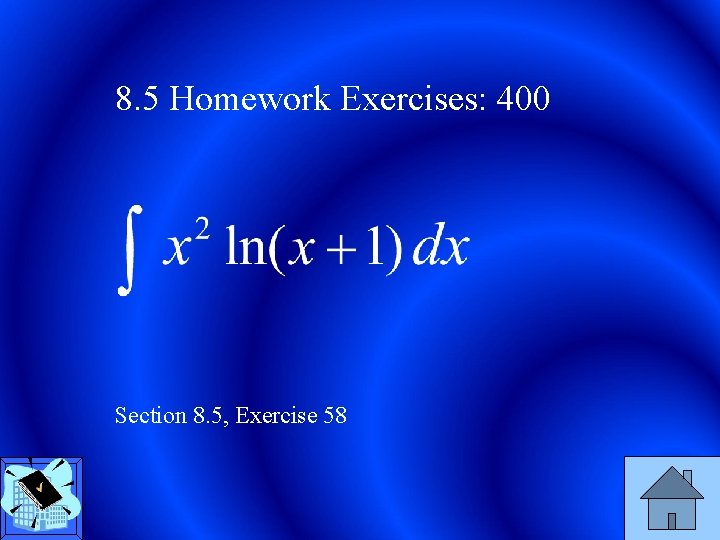8. 5 Homework Exercises: 400 Section 8. 5, Exercise 58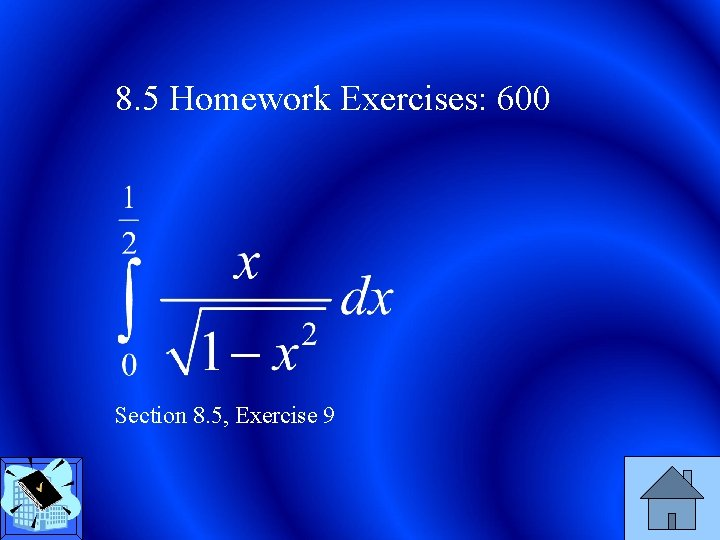8. 5 Homework Exercises: 600 Section 8. 5, Exercise 9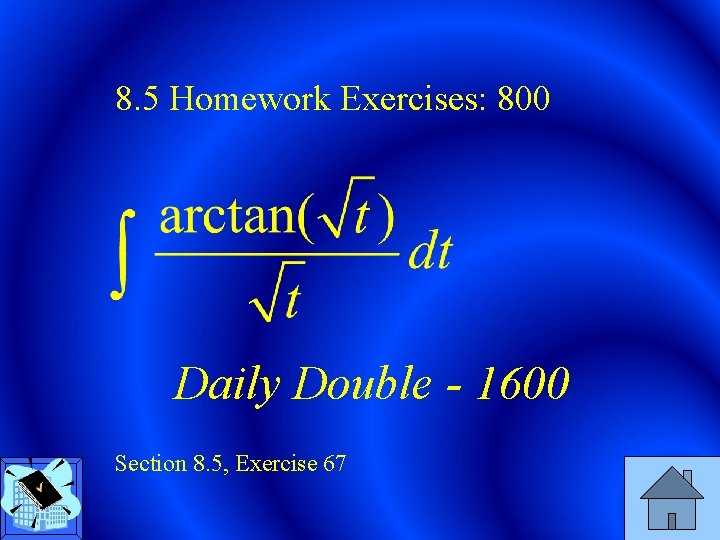8. 5 Homework Exercises: 800 Daily Double - 1600 Section 8. 5, Exercise 67Potpourri: 200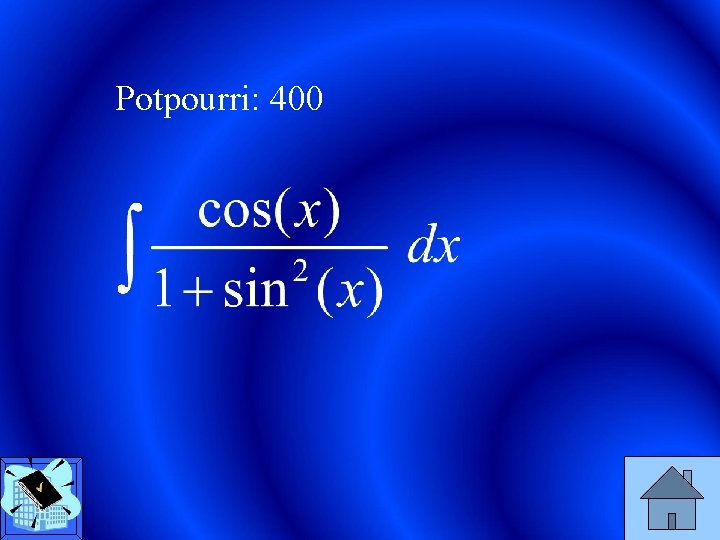Potpourri: 400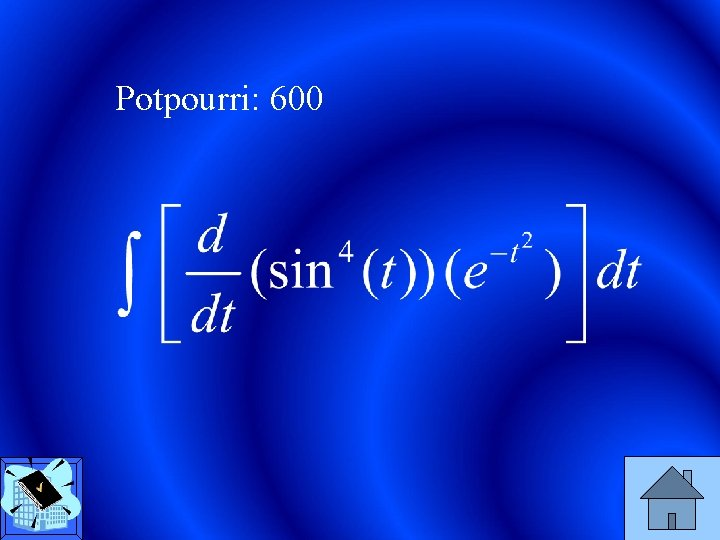Potpourri: 600Potpourri: 800n Integration by Substitution: 200 o ti u l So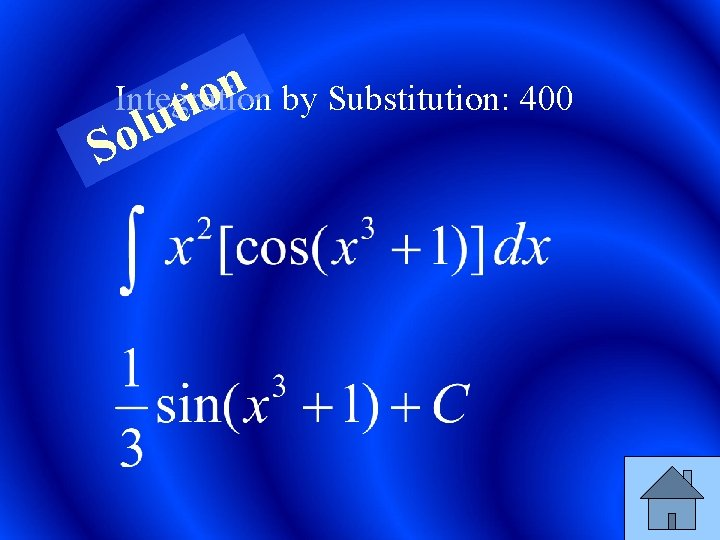n Integration by Substitution: 400 o ti u l Son Integration by Substitution: 600 o ti u l So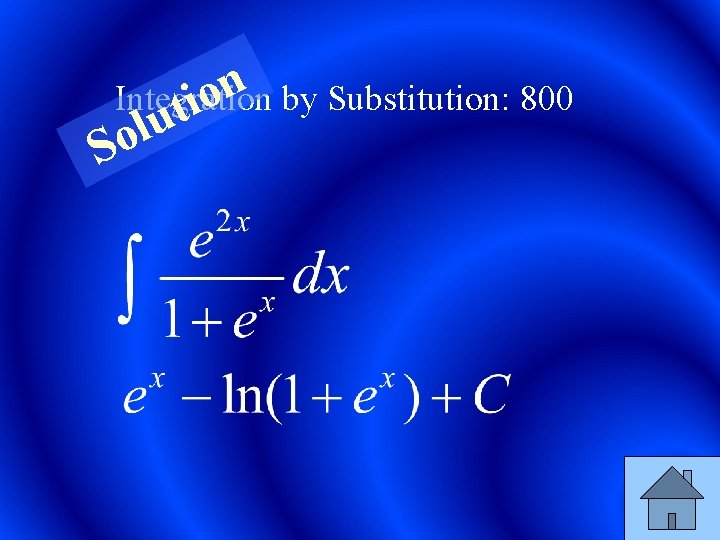n Integration by Substitution: 800 o ti u l So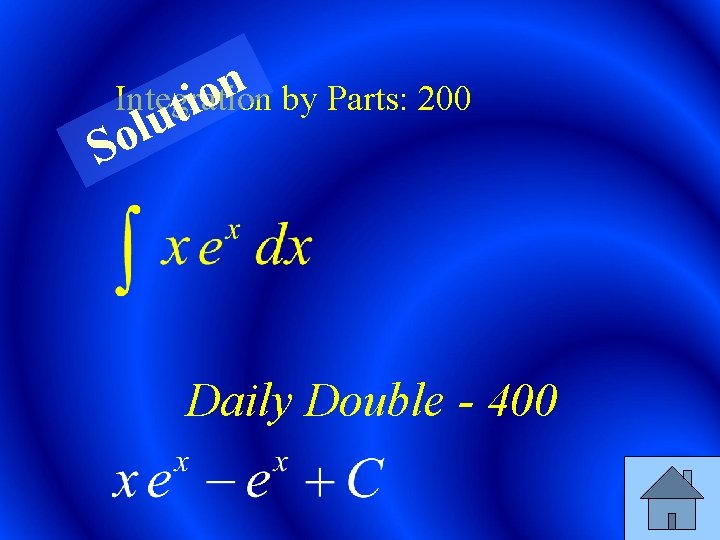n Integration by Parts: 200 o ti u l So Daily Double - 400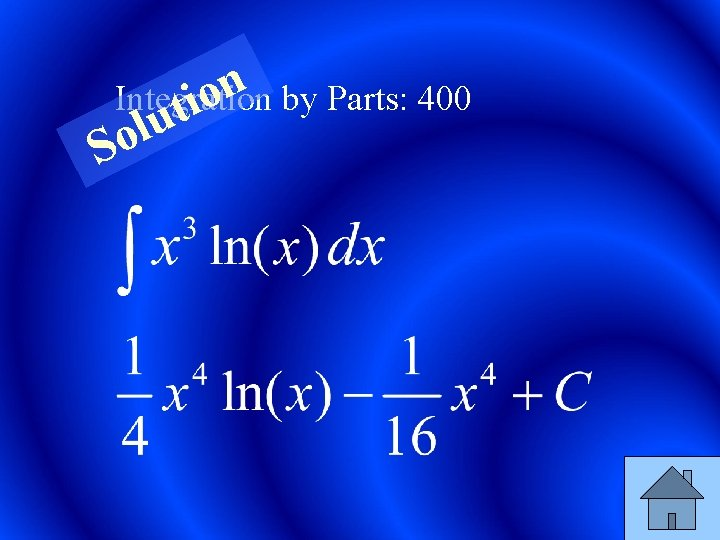n Integration by Parts: 400 o ti u l Son Integration by Parts: 600 o ti u l Son Integration by Parts: 800 o ti u l So Daily Double - 1600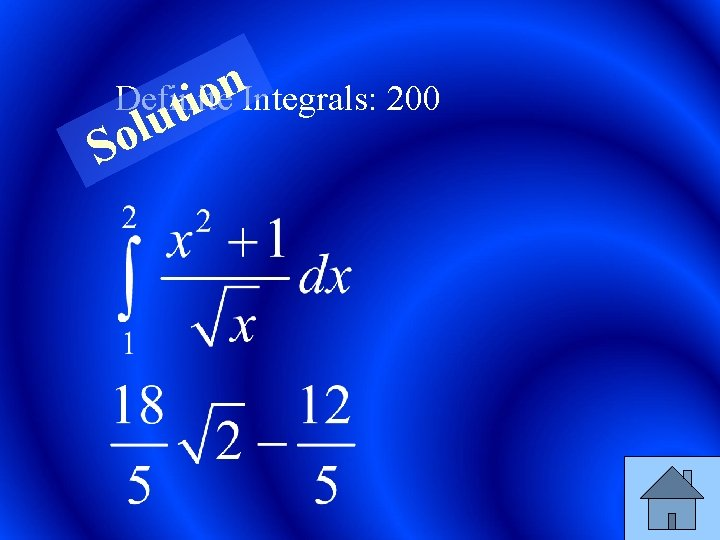n Definite o ti Integrals: 200 u l Son Definite o ti Integrals: 400 u l Son Definite o ti Integrals: 600 u l Son Definite o ti Integrals: 800 u l So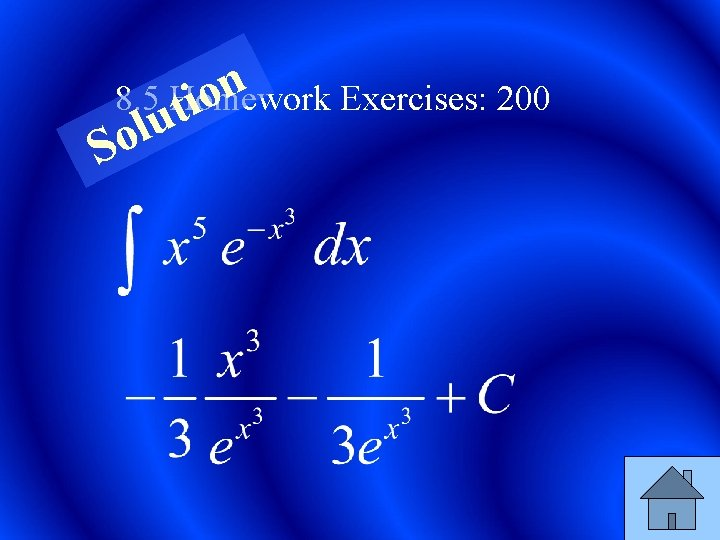n 8. 5 Homework Exercises: 200 o ti u l So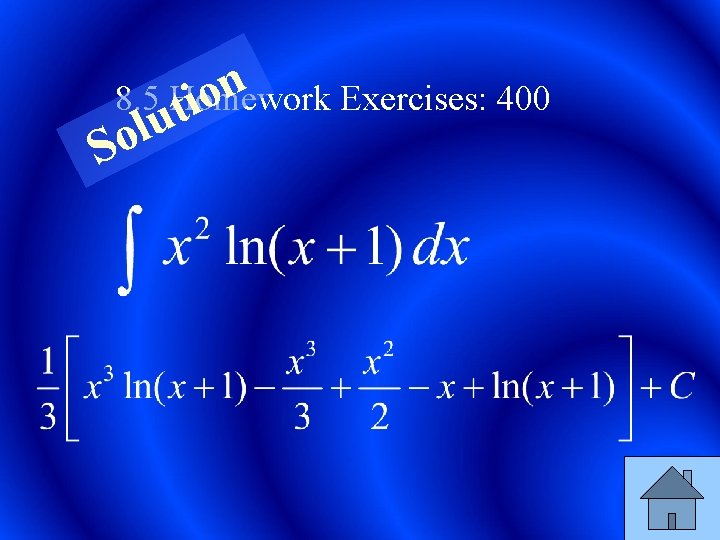n 8. 5 Homework Exercises: 400 o ti u l Son 8. 5 Homework Exercises: 600 o ti u l Son 8. 5 Homework Exercises: 800 o ti u l So Daily Double - 1600n Potpourri: o ti 200 u l Son Potpourri: o ti 400 u l Son Potpourri: o ti 600 u l Son Potpourri: o ti 800 u l So